# Philosophy, Computing, and Artificial Intelligence

PHI 319. Recognizing Digits in the MNIST Data Set.

## Artificial Neural Networks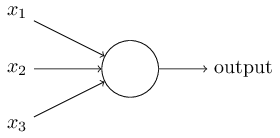A neuron processes and transmits information. In the human brain, there are about 85 billion neurons. A typical neuron has dendrites, a cell body, and an axon. The dendrites (from the Greek δενδρίτης) take input from other neurons in the form of electrical impulses. The cell body processes these inputs, and the axon terminals transmit outputs in the form of electrical impulses.

Artificial neural networks are computational models of neural networks in the brain.

## Perceptron Neurons

An artificial neuron is a computational model of a neuron. A perceptron takes binary inputs (xj) and computes a binary output. The computation involves weights (wj) and a threshold value. If Σj wj xj is greater than the threshold value, then the output is 1. Otherwise, it is 0. Perceptrons can implement logic functions. Conjunction (φ ∧ ψ) is an example. Let the perceptron have two inputs, a weight of 0.6 each, and a threshold value of 1. If both inputs are 1, the sum exceeds the threshold and thus the output is 1. Otherwise, the output is 0. These conditions for activating the perceptron match the truth-table for conjunction (∧).

This is an instance of the integrate-and-fire model. A neuron receives its inputs through its synapses. Because the weighs correspond to the relative efficiency with which a synapse communicates inputs to the cell body, some inputs weigh more heavily than others in the computation. Since it takes resources for the neuron to fire, the neuron is quiet unless the threshold is crossed.

The computation in a perceptron is typically expressed mathematically as the dot product w · x (= wTx = Σj wj xj), where w is a m-vector of weights and x is a m-vector of inputs. The negative of the threshold value is the perceptron's bias, b. In these terms, the value of the output activation function for a given set of inputs is 1 if w · x + b > 0 and is 0 otherwise.Artificial neurons may be linked together in a feedfoward network in which the ouput from one layer is the input to the next layer. The first layer is input layer of neurons. The last layer is the output layer. The hidden layers are the neurons that are neither input nor output neurons.

A feedforward network of artificial neurons may be understood as a device that makes "decisions about decisions." The first layer of neurons makes a "decision" by weighing the input, the next layer makes a "decision about the decision" of the prior layer, and so on.

## Sigmoid Neurons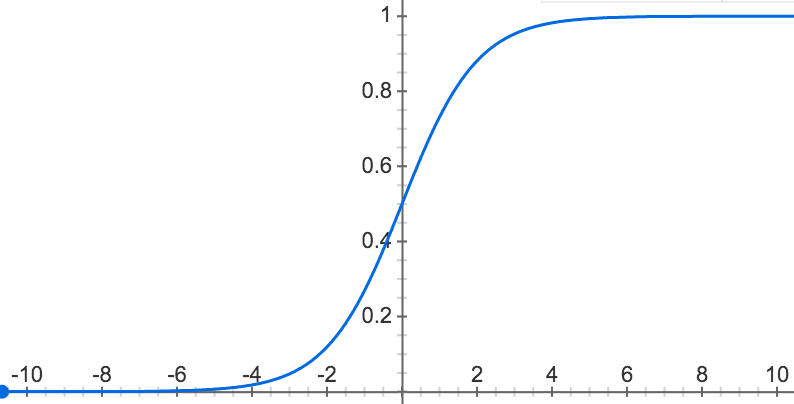A sigmoid neuron has an important feature a perceptron lacks: small changes in the weights and bias cause only small changes in the output. This allows sigmoid neurons to "learn."

A sigmoid neuron has the same mathematical parts as a perceptron (inputs, weights, and a bias), but the inputs may have any value between 0 and 1. The output is not binary either. Instead, it is f(w · x + b), where the activation function f is the sigmoid function.

The sigmoid function is σ(x) =  1/(1 + e^-x)

As the activation function, the sigmoid function maps w · x + b to a smooth curve that preserves desirable features of the activation function for perceptrons. When w · x + b is a large positive number, the output of the function is close to 1 because e^-x is close to 0. When w · x + b is a large negative number, the output is close to 0 because e^-x is close to infinity.

## A Network to Classify Handwritten DigitsThe MNIST data set contains scanned images of handwritten digits. (MNST is a modified subset of two data sets collected by the National Institute of Standards and Technology (NIST).)

The images are greyscale and 28 by 28 pixels in size.

They are split into 60,000 training images and 10,000 test images.

The input to each neuron in the input layer in the network is one pixel from the input image. Since each image is 28 x 28 pixels, the input layer has 784 (or 28 x 28) neurons.

In the original MNIST data set, the images are coded in greyscale (where 0 is black, 255 is white, and values in between black and white are decreasing shades of gray).

To make the data set convenient to use in Python, an image takes the form of a NumPy (the package for scientific computing with Python) one-dimensional array of 784 values between 0 and 1 (where 0 is black, 1 is white, and values in between are decreasing shades of gray).

The output layer in the network has 10 neurons. The first neuron in this layer indicates whether the image is a 0, the second whether the image is a 1, and so on.

### Minimizing the Error Function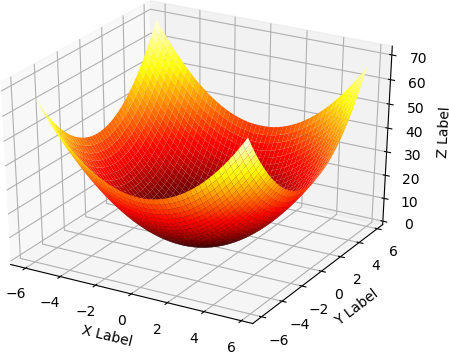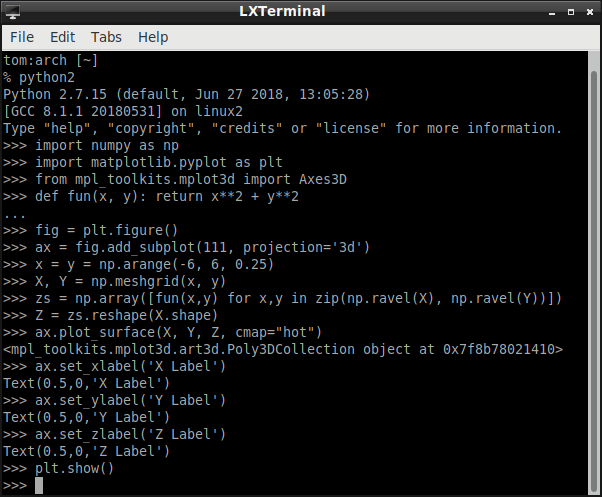This network needs to be "trained" to classify the digits correctly.

The amount of error in a network is sensitive to the weights and biases. Training a network is a matter of finding weights and biases that minimize the error.

To get some insight into the general idea, consider the functionf(x,y) = x^2 + y^2.

The gradient of this function is the vector of partial derivatives

gradf(x,y) = [[(delf)/(delx)(x,y)], [(delf)/(dely)(x,y)]] = [[2x],[2y]] .

This vector points in the direction the function increases most rapidly. If the starting-point is (1,3), the direction of steepest ascent is toward

gradf(1,3) = [,]

If we step in the direction of steepest descent from (1,3) with step size eta = 0.1, we arrive at (0.8,2.4). (In the x direction, from 1 we step -0.1*2. In the y direction, from 3 we step -0.1*6.) If we step down again, we arrive at (0.64,1.92). If we keep descending, we approach (0,0).

### An Example Image from the MNIST Data Set

The image below of the handwritten numeral "5" is in training_data, which is a list of 50,000 2-tuples (x, y). The x is a 784-dimensional array containing the input image. The y is a 10-dimensional array corresponding to the label for image.training_data is the x in the first tuple. training_data is the y in the first tuple.


tom:arch [~/git/neural-networks-and-deep-learning/src]
% python2
Python 2.7.12 (default, Jun 28 2016, 08:31:05)
[GCC 6.1.1 20160602] on linux2
Type "help", "copyright", "credits" or "license" for more information.
>>> import mnist_loader
>>> training_data, validation_data, test_data = mnist_loader.load_data_wrapper()
>>> training_data.shape
(10, 1)
>>> training_data
array([[ 0.],
[ 0.],
[ 0.],
[ 0.],
[ 0.],
[ 1.],
[ 0.],
[ 0.],
[ 0.],
[ 0.]])
>>> training_data.shape
(784, 1)
>>> import numpy as np
>>> image_array = np.reshape(training_data, (28, 28))
>>> import matplotlib.pyplot as plt
>>> image = plt.imshow(image_array, cmap ='gray')
>>> plt.show()	

## mnist_loader.py

The data set is from a tutorial on the site Deep Learning. The file is a "pickled" tuple of three lists. Each of the three lists is formed from a list of images and list of labels. An image is represented as NumPy one-dimensional array of 784 float values between 0 and 1 (0 for black, 1 for white). The labels are numbers between 0 and 9 indicating which digit the image represents.

The function load_data_wrapper() returns training_data, validation_data, test_data.

validation_data and test_data are lists containing 10,000 2-tuples (x, y). x is a 784-dimensional array containing the input image. y is the label for the image.


import cPickle   # Python object serialization
import gzip
import numpy as np

def load_data():
f = gzip.open('../data/mnist.pkl.gz', 'rb')
training_data, validation_data, test_data = cPickle.load(f)
f.close()
return (training_data, validation_data, test_data)

def load_data_wrapper():
tr_d, va_d, te_d = load_data()
training_inputs = [np.reshape(x, (784, 1)) for x in tr_d]
training_results = [vectorized_result(y) for y in tr_d]
training_data = zip(training_inputs, training_results)
validation_inputs = [np.reshape(x, (784, 1)) for x in va_d]
validation_data = zip(validation_inputs, va_d)
test_inputs = [np.reshape(x, (784, 1)) for x in te_d]
test_data = zip(test_inputs, te_d)
return (training_data, validation_data, test_data)

def vectorized_result(j):
e = np.zeros((10, 1))
e[j] = 1.0
return e

## The Rest of the Python Program

We will not try to understand the program code (which belongs to Michael Nielsen) or the underlying algorithm in complete detail.

### The Network Class

class Network(object):

def __init__(self, sizes):
self.num_layers = len(sizes)
self.sizes = sizes
self.biases = [np.random.randn(y, 1) for y in sizes[1:]]
self.weights = [np.random.randn(y, x) for x, y in zip(sizes[:-1], sizes[1:])]

We can use this class to create a neural network. (Python is an object-oriented programming language.) So, for example, the instruction

net = network.Network([2, 3, 1])

creates a neural network (net) whose input layer has two neurons, whose middle layer has three neurons, and whose output layer has one neuron.

 tom:arch [~/git/neural-networks-and-deep-learning/src] % python2 Python 2.7.12 (default, Jun 28 2016, 08:31:05) [GCC 6.1.1 20160602] on linux2 Type "help", "copyright", "credits" or "license" for more information. >>> import network # import module >>> net = network.Network([2, 3, 1]) # create instance of class >>> >

The biases and weights are set as random numbers. The input layer has no bias. Biases are only used in computing the output from later layers.

For the [2, 3, 1] network, the biases are in a 3 x 1 array and a 1 x 1 array.

tom:arch [~/git/neural-networks-and-deep-learning/src]
% python2
Python 2.7.12 (default, Jun 28 2016, 08:31:05)
[GCC 6.1.1 20160602] on linux2
Type "help", "copyright", "credits" or "license" for more information.
>>> import network
>>> net = network.Network([2, 3, 1])
>>> net.biases.shape
(3, 1)
>>> net.biases
array([[ 1.36630966],
[ 1.05788544],
[ 0.80606255]])
>>>net.biases.shape
(1, 1)
>>>net.biases
array([[ 1.54813682]])
>>>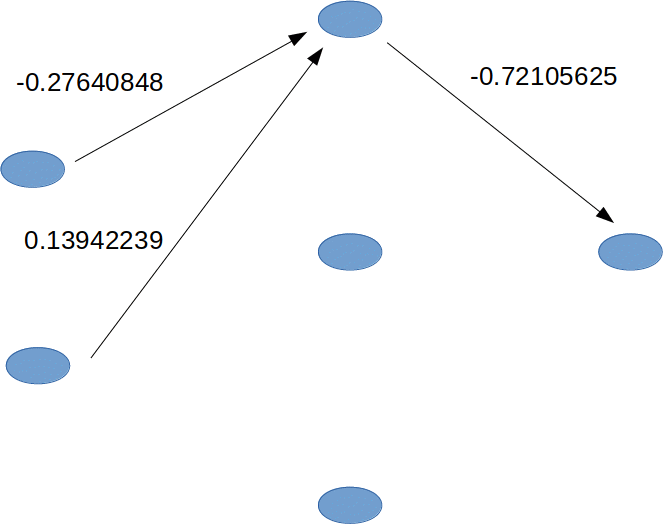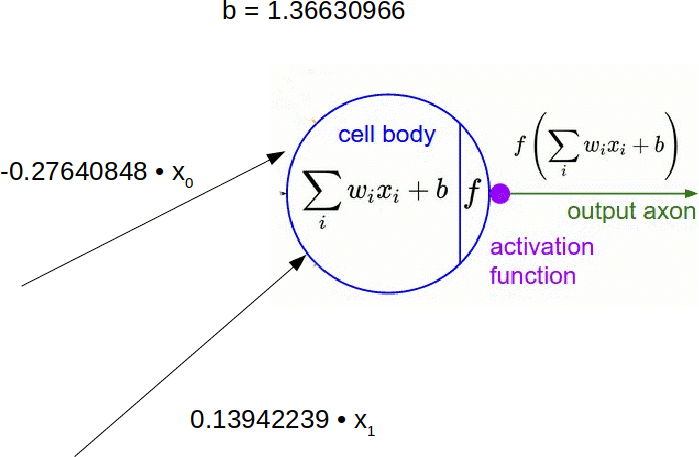For the [2, 3, 1] network, the weights are in a 3 x 2 array and a 1 x 3 array.

The first row in net.weights are the respective weights the first neuron in the hidden layer attributes to the outputs of the first and second neurons in the input layer.

>>> net.weights.shape
(3, 2)
>>> net.weights
array([[-0.27640848,  0.13942239],
[ 1.13350606,  1.51767629],
[-0.03836741,  0.06409297]])
>>> net.weights.shape
(1, 3)
>>> net.weights
array([[-0.72105625,  1.76366748,  1.49408987]])
>>> 

### Stochastic (Mini-Batch) Gradient Descent

For each "epoch" of training, the training data is randomly shuffled and partitioned into mini-batches. Once the last mini-batch is processed, the network is evaluated against the test data.

def SGD(self, training_data, epochs, mini_batch_size, eta,
test_data=None):
if test_data: n_test = len(test_data)
n = len(training_data)
for j in xrange(epochs):
random.shuffle(training_data)
mini_batches = [training_data[k:k+mini_batch_size] for k in xrange(0, n, mini_batch_size)]
for mini_batch in mini_batches:
self.update_mini_batch(mini_batch, eta)
if test_data
print "Epoch {0}: {1} / {2}".format(j, self.evaluate(test_data), n_test)
else:
print "Epoch {0} complete".format(j)	

The method update_mini_batch updates the weights and biases. For each input in the mini-batch, it calculates the adjustments to the weights and biases that reduce the value of the error function. Given the learning rate and the average of the adjustments to the weights and biases for the inputs in the mini-batch, it updates the weights and biases in the network.

	def update_mini_batch(self, mini_batch, eta):
nabla_b = [np.zeros(b.shape) for b in self.biases]
nabla_w = [np.zeros(w.shape) for w in self.weights]
#
#
#
for x, y in mini_batch:
delta_nabla_b, delta_nabla_w = self.backprop(x, y)
nabla_b = [nb+dnb for nb, dnb in zip(nabla_b, delta_nabla_b)]
nabla_w = [nw+dnw for nw, dnw in zip(nabla_w, delta_nabla_w)]
#
# update weights and biases
#
self.weights = [w-(eta/len(mini_batch))*nw for w, nw in zip(self.weights, nabla_w)]
self.biases = [b-(eta/len(mini_batch))*nb for b, nb in zip(self.biases, nabla_b)]	

The update_mini_batch uses the method backprop to compute the adjustments.

The backprop method has two parts. In #feedforward, it forward feeds the training input (x) through the network. It stores the zs and activations layer by layer. It uses the zs and activations in #backward pass to calculate the adjustments.

def backprop(self, x, y):
#
# x is the input to the network, y is the label for x
#
nabla_b = [np.zeros(b.shape) for b in self.biases]
nabla_w = [np.zeros(w.shape) for w in self.weights]
#
# feedforward
# feed x forward through the network
#
activation = x
activations = [x]
zs = []
for b, w in zip(self.biases, self.weights):
z = np.dot(w, activation)+b
zs.append(z)
activation = sigmoid(z)
activations.append(activation)
#
# The first time through the loop the activation is the input to the network
# and w and b are the weights and biases the second layer imposes on this input.
#
# In the [784, 30, 10] MNIST network, the input is a 784x1 array and w and b are a 30x784
# array and a 30x1 array. The 30x1 array of weighted sums input to the second layer is
# stored in zs. The 30x1 array of outputs of the second layer is stored in activations.
#
#
# fundamental equation 1 (* is the Hadamard product)
delta = self.cost_derivative(activations[-1], y) * sigmoid_prime(zs[-1])
#
# fundamental equation 3
nabla_b[-1] = delta
#
# fundamental equation 4
nabla_w[-1] = np.dot(delta, activations[-2].transpose())
#
for l in xrange(2, self.num_layers):
z = zs[-l]
sp = sigmoid_prime(z)
delta = np.dot(self.weights[-l+1].transpose(), delta) * sp               # fundamental equation 2
nabla_b[-l] = delta                                                      # fundamental equation 3
nabla_w[-l] = np.dot(delta, activations[-l-1].transpose())               # fundamental equation 4
#
#
# return the adjustments
#
return (nabla_b, nabla_w)

def cost_derivative(self, output_activations, y):
return (output_activations-y) 

When an image x from the training set of n images is fed through the network, it produces a vector of outputs, a, different in general from the desired vector of outputs y(x). The ideal is to make y(x) = a for all the images in the training set. We want to minimize the error

E = 1/(2n) sum_x^n norm(y(x) - a)^2

Q: Why square the L2 (Euclidean) norm?
A: The choice of error functions depends on several issues. In part, it depends on how deviations from the target are valued.
Squaring the norm penalizes large deviations more than small deviations.
Q: Why the constant 1/2?
A: To cancel the exponent when differentiating.
Since we set the learning rate, the introduction of a constant does not matter.

The derivative of the error function for a given input to the network with respect to the activation of a neuron in the output layer is

(delE)/(dela_L^k) = del/(dela_L^k)[1/2(y-a_L^k)^2]
= 1/2 * del/(dela_L^k)[(y-a_L^k)^2]
= 1/2 * 2(y-a_L^k) * del/(dela_L^k)[y-a_L^k]
= (del/(dela_L^k)y - del/(dela_L^k)a_L^k) * (y-a_L^k)

 = (0 -1) * (y-a_L^k) = -(y-a_L^k)
(delE)/(dela_L^k) = a_L^k-y

def sigmoid(z):
return 1.0/(1.0+np.exp(-z))

def sigmoid_prime(z):
# derivative of the sigmoid function
return sigmoid(z)*(1-sigmoid(z))

The evaluate method returns the number of test inputs for which the network output is correct.

def evaluate(self, test_data):
# the output is the index of the first neuron in the final layer with a maximum activation
test_results = [(np.argmax(self.feedforward(x)), y) for (x, y) in test_data]
return sum(int(x == y) for (x, y) in test_results)

def feedforward(self, a):
for b, w in zip(self.biases, self.weights):
a = sigmoid(np.dot(w, a)+b)
return a	  

## The Four Fundamental Equations

delta_l^j (the error in the j^(th) neuron in the l^(th) layer) is defined as (delE)/(delz_l^j), where

z_l^j = sum_k w_l^(jk) a_(l-1)^k + b_l^j, for neuron k in the (l-1)^(th) layer

w_l^(jk) is the weight from the k^(th) neuron in the (l - 1)^(th) layer to the j^(th) neuron in the l^(th) layer

a_l^j = sigma(sum_k w_l^(jk) a_(l-1)^k + b_l^j), for neuron k in the (l-1)^(th) layer

b_l^j is the bias of the j^(th) neuron in the l^(th) layer

Fundamental equation 1: delta_L^j = (delE)/(dela_L^j)sigma'(z_L^j).

Since a_L^j = sigma(z_L^j), (delE)/(dela_L^j)sigma'(z_L^j) = (delE)/(dela_L^j) (dela_L^j)/(delz_L^j).   By the chain rule, (delE)/(dela_L^j) (dela_L^j)/(delz_L^j) = (delE)/(delz_L^j).

Fundamental equation 2: delta_l^j = sum_k w_(l+1)^(jk)delta_(l+1)^ksigma'(z_l^j).

By definition and the chain rule, delta_l^j = (delE)/(delz_l^j) = sum_k (delz_(l+1)^k)/(delz_l^j) (delE)/(delz_(l+1)^k) = sum_k (delz_(l+1)^k)/(delz_l^j) delta_(l+1)^k

Since z_(l+1)^k = sum_m w_(l+1)^(mk)a_l^m + b_(l+1)^k = sum_m w_(l+1)^(mk)sigma(z_l^m) + b_(l+1)^k, it follows that (delz_(l+1)^k)/(delz_l^j) = w_(l+1)^(jk)sigma'(z_l^j)

Fundamental equation 3: (delE)/(delb_l^j) = delta_l^j.

By the chain rule and definition, (delE)/(delb_l^j) = (delE)/(delz_l^j) (delz_l^j)/(delb_l^j) = delta_l^j(delz_l^j)/(delb_l^j). Since z_l^j = sum_k w_l^(jk)a_(l-1)^j + b_l^j, it follows that (delz_l^j)/(delb_l^j) = 1

Fundamental equation 4: (delE)/(delw_l^(jk)) = a_(l-1)^kdelta_l^j.

By the chain rule and definition, (delE)/(delw_l^(jk)) = (delz_l^j)/(delw_l^(jk)) (delE)/(delz_l^j) = (delz_l^j)/(delw_l^(jk))delta_l^j. Since, z_l^j = sum_k w_l^(jk)a_(l-1)^j + b_l^j, it follows that (delz_l^j)/(delw_l^(jk)) = a_(l-1)^j

## The [784,30,10] Network in Action

The network has 784 neurons in the input layer, 30 in the hidden layer, and 10 in the output layer. The code to train the network uses mini-batch, stochastic gradient descent to learn from the MNIST training_data over 30 epochs. The mini-batch size is 10. The learning rate (η) is 3.0. After the network has been trained, the code tests the network with a random image.

tom:arch [~/git/neural-networks-and-deep-learning/src]
% python2
Python 2.7.12 (default, Nov  7 2016, 11:55:55)
[GCC 6.2.1 20160830] on linux2
Type "help", "copyright", "credits" or "license" for more information.
>>> import mnist_loader
>>> training_data, validation_data, test_data = mnist_loader.load_data_wrapper()
>>> import network
>>> net = network.Network([784, 30, 10])
>>> net.SGD(training_data, 30, 10, 3.0, test_data=test_data)
Epoch 0: 8268 / 10000
Epoch 1: 8393 / 10000
Epoch 2: 8422 / 10000
Epoch 3: 8466 / 10000

.
.
.
Epoch 27: 9497 / 10000
Epoch 28: 9495 / 10000
Epoch 29: 9478 / 10000
>>> import numpy as np
>>> imgnr = np.random.randint(0,10000)
>>> prediction = net.feedforward( test_data[imgnr] )
>>> print("Image number {0} is a {1}, and the network predicted a {2}".format(imgnr, test_data[imgnr], np.argmax(prediction)))
Image number 4709 is a 2, and the network predicted a 2
>>> import matplotlib.pyplot as plt
>>> fig, ax = plt.subplots(1,2,figsize=(8,4))
>>> ax.matshow( np.reshape(test_data[imgnr], (28,28) ), cmap='gray' )
>>> ax.plot( prediction, lw=3 )
>>> ax.set_aspect(9)
>>> plt.show()


The trained network predicts that the image (chosen randomly) is a "2."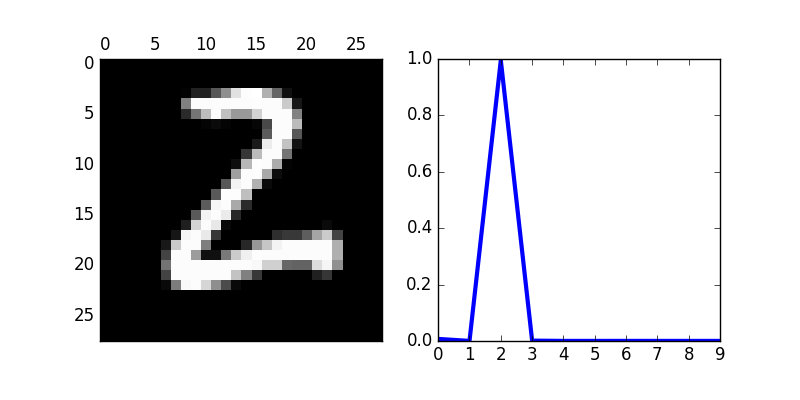## Convolutional Neural Networks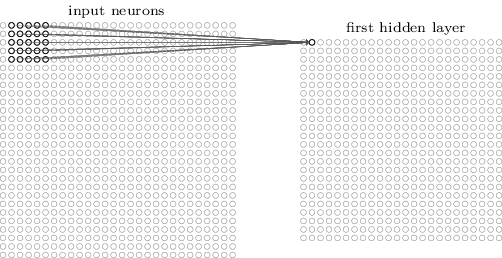These images come from the explanation of convolutional neural networks in Neural Networks and Deep Learning.
The layers in a convolutional neural networks are not fully-connected. (This allows them to be sensitive to spatial structure.) Instead, they have convolutional layers.

Each neuron in the first hidden layer is connected to a small region of the input image. This is the local receptive field. The next neuron in the hidden layer is connected to a local receptive field that overlaps with the previous field. The extent of the overlap is determined by the stride length.

The size of the input and the local receptive field determines the size of the first hidden layer. For 28 x 28 input, 5 x 5 local receptive field, and stride of 1, the first hidden layer is 24 x 24.

Each hidden neuron has a bias and a set of weights. If the local receptive field is 5 x 5, then the hidden neuron has a 5 x 5 set of weights. Moreover, the biases and weights are the same for each neuron in the hidden layer. So the neurons in the first hidden layer detect the same input pattern or feature, no matter where it is in the image.

We can think of the hidden layer as consisting of a set of feature maps. If a feature map is 24 x 24, then a hidden layer consisting of 2 x 24 x 24 neurons consists in two maps and can detect two features.

There are different forms of pooling. In max pooling, the pooling neuron outputs the maximum of the region in the feature map. In L2 pooling, the neuron outputs the square root of the sum of the squares of the activations in the region. Convolutional neural networks also have pooling layers.

Pooling layers summarize the information in a region of a feature map. If the hidden layer is 24 x 24, and the region to summarize is 2 x 2, then the pooling layer is 12 x 12.

Conv architecture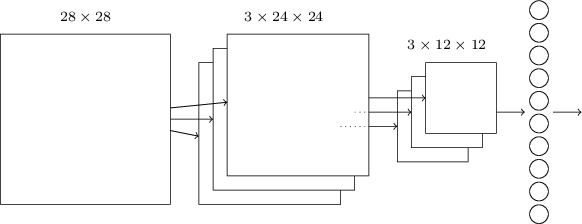Conv + FC architecture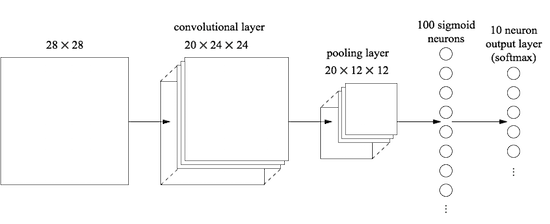In the first example (Conv architecture), the input is 28 x 28. The next layer in the network is the convolutional layer. It uses a 5 x 5 local receptive field and 3 feature maps. So the convolutional layer is 3 x 24 x 24. The pooling layer is next in the network. The size of the region summarized in the feature maps is 2 x 2. So the pooling layer is 3 x 12 x 12. The final layer is fully-connected. Every neuron in the pooling layer is connected to everyone one of the 10 output neurons.

In the second example (Conv + FC architecture), the convolutional nueral network is more complicated. There are 20 feature maps. In addition, the output layer is a softmax layer. Further, there is a fully-connected layer between the pooling layer and the softmax layer. In a softmax layer, the softmax function (not the sigmoid function) is applied to get the activation. The output of the softmax function is a probability distribution. So a_L^j is the probability that the digit the image represents is j.

### The Python/Theano Program

In the following session, the convolutional neural network (net) has the "Conv + FC architecture" represented in the second example. The input is a 28 x 28 image from the MNIST dataset. The convolutional layer is 20 x 24 x 24. The pooling layer is 3 x 12 x 12. These layers are followed by a fully-connected layer and a softmax output layer.

% python2
Python 2.7.12 (default, Nov  7 2016, 11:55:55)
[GCC 6.2.1 20160830] on linux2
Type "help", "copyright", "credits" or "license" for more information.
>>> import network3_tab
>>> from network3_tab import Network
>>> from network3_tab import ConvPoolLayer, FullyConnectedLayer, SoftmaxLayer
>>> training_data, validation_data, test_data = network3_tab.load_data_shared()
>>> mini_batch_size = 10
>>> net = Network([
...      ConvPoolLayer(image_shape=(mini_batch_size, 1, 28, 28), filter_shape=(20, 1, 5, 5)),
...      FullyConnectedLayer(n_in=20*12*12, n_out=100),
...      SoftmaxLayer(n_in=100, n_out=10)],
...      mini_batch_size)
>>> 

### Load the MNIST data

The MNIST data is pickled as a tuple of three lists. Each of the three lists is formed from a list of images and list of labels. The images and labels are stored in Theano shared variables so that the calculations can be processed on the GPU. In GPU memory, the data must be stored as a floating point. The program uses the labels as integers, so shared_y is returned as an integer.

def load_data_shared(filename="../data/mnist.pkl.gz"):
f = gzip.open(filename, 'rb')
training_data, validation_data, test_data = cPickle.load(f)
f.close()
def shared(data):
shared_x = theano.shared(
np.asarray(data, dtype=theano.config.floatX), borrow=True)
shared_y = theano.shared(
np.asarray(data, dtype=theano.config.floatX), borrow=True)
return shared_x, T.cast(shared_y, "int32")
return [shared(training_data), shared(validation_data), shared(test_data)]	

### The ConvPoolLayer, FullyConnectedLayer, and SoftmaxLayer

The first layer in net is really two layers: a convolutional layer and a max-pooling layer.

ConvPoolLayer initializes the weights using a Gaussian distribution with mean 0 and standard deviation 1 over the square root of the number of weights connecting to the same neuron. (This helps prevent saturation.) It initializes the biases using a Gaussian distribution with mean 0 and standard deviation 1. It loads these weights and biases into shared variables. The method set_inpt defines the algorithm for symbolically calculating the output of the layer. It uses theano.tensor.nnet.conv2d and theano.tensor.signal.pool.pool_2d. (Convolution arithmetic tutorial)

class ConvPoolLayer(object):
def __init__(self, filter_shape, image_shape, poolsize=(2, 2), activation_fn=sigmoid):
self.filter_shape = filter_shape
self.image_shape = image_shape
self.poolsize = poolsize
self.activation_fn=activation_fn
# initialize weights and biases
n_out = (filter_shape*np.prod(filter_shape[2:])/np.prod(poolsize))
self.w = theano.shared(
np.asarray(
np.random.normal(loc=0, scale=np.sqrt(1.0/n_out), size=filter_shape),
dtype=theano.config.floatX),
borrow=True)
self.b = theano.shared(
np.asarray(
np.random.normal(loc=0, scale=1.0, size=(filter_shape,)),
dtype=theano.config.floatX),
borrow=True)
self.params = [self.w, self.b]

def set_inpt(self, inpt, mini_batch_size):
self.inpt = inpt.reshape(self.image_shape)
conv_out = conv.conv2d(
input=self.inpt, filters=self.w, filter_shape=self.filter_shape,
image_shape=self.image_shape)
pooled_out = pool.pool_2d(
input=conv_out, ds=self.poolsize, ignore_border=True)
self.output = self.activation_fn(
pooled_out + self.b.dimshuffle('x', 0, 'x', 'x'))

The other two layer classes (FullyConnectedLayer and SoftmaxLayer) are similar to ConvPoolLayer. The primary difference is in the set_inpt method.

class FullyConnectedLayer(object):
def __init__(self, n_in, n_out, activation_fn=sigmoid):
self.n_in = n_in
self.n_out = n_out
self.activation_fn = activation_fn
# Initialize weights and biases
self.w = theano.shared(
np.asarray(
np.random.normal(
loc=0.0, scale=np.sqrt(1.0/n_out), size=(n_in, n_out)),
dtype=theano.config.floatX),
name='w', borrow=True)
self.b = theano.shared(
np.asarray(np.random.normal(loc=0.0, scale=1.0, size=(n_out,)),
dtype=theano.config.floatX),
name='b', borrow=True)
self.params = [self.w, self.b]

def set_inpt(self, inpt, mini_batch_size):
self.inpt = inpt.reshape((mini_batch_size, self.n_in))
self.output = self.activation_fn(
T.dot(self.inpt, self.w) + self.b)
self.y_out = T.argmax(self.output, axis=1)

def accuracy(self, y):
return T.mean(T.eq(y, self.y_out))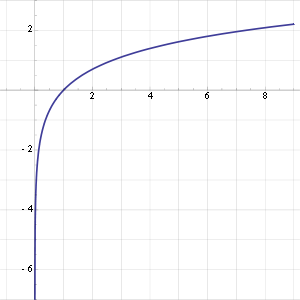The cost function in SoftmaxLayer is the negative log-likelihood function.

If x is the input to the network and y is the desired output, then the log-likelihood cost of x is -ln a_L^j. As the probability of output approaches 1, the cost approaches 0. As the probability of the output approaches 0, the cost approaches infinity.


class SoftmaxLayer(object):
def __init__(self, n_in, n_out):
self.n_in = n_in
self.n_out = n_out
# Initialize weights and biases
self.w = theano.shared(
np.zeros((n_in, n_out), dtype=theano.config.floatX),
name='w', borrow=True)
self.b = theano.shared(
np.zeros((n_out,), dtype=theano.config.floatX),
name='b', borrow=True)
self.params = [self.w, self.b]

def set_inpt(self, inpt, mini_batch_size):
self.inpt = inpt.reshape((mini_batch_size, self.n_in))
self.output = softmax(T.dot(self.inpt, self.w) + self.b)
self.y_out = T.argmax(self.output, axis=1)

def cost(self, net):
# net.y.shape is the number of the training examples in the minibatch (N)
# T.arange(net.y.shape) is a symbolic vector of integers [0,1,2,...,N-1]
# T.log(self.output) is a NxK matrix, where K = 10 (the number of digits 0..9)
# T.log(self.output)[T.arange(net.y.shape), net.y] is a vector of length N
# with the log-likelihoods of the labels
# The mean is the average across the all the training examples in the minibatch
return -T.mean(T.log(self.output)[T.arange(net.y.shape), net.y])

def accuracy(self, y):
return T.mean(T.eq(y, self.y_out))

### The Network Class

The Network class creates a network from a list of layers and a minibatch size. It defines the symbolic variables for the input (self.x) to and desired output (self.y) from the network. It sets the input to the initial layer. It propagates self.x forward through the layers of the network in to symbolically define the output from the network.

The method SGD trains the network using mini-batch stochastic gradient descent. The functions train_mb and test_mb_accuracy are called in the training.

class Network(object):

def __init__(self, layers, mini_batch_size):
self.layers = layers
self.mini_batch_size = mini_batch_size
self.params = [param for layer in self.layers for param in layer.params]
self.x = T.matrix("x")
self.y = T.ivector("y")
init_layer = self.layers
init_layer.set_inpt(self.x, self.mini_batch_size)
for j in xrange(1, len(self.layers)):
prev_layer, layer  = self.layers[j-1], self.layers[j]
layer.set_inpt(prev_layer.output, self.mini_batch_size)
self.output = self.layers[-1].output

def SGD(self, training_data, epochs, mini_batch_size, eta, test_data):
training_x, training_y = training_data
test_x, test_y = test_data
num_training_batches = size(training_data)/mini_batch_size
num_test_batches = size(test_data)/mini_batch_size
cost = self.layers[-1].cost(self)
grads = T.grad(cost, self.params)
updates = [(param, param-eta*grad) for param, grad in zip(self.params, grads)]
# define functions to train a mini-batch compute the accuracy in test mini-batches.
i = T.lscalar() # mini-batch index
train_mb = theano.function(
[i], cost, updates=updates,
givens={
self.x:
training_x[i*self.mini_batch_size: (i+1)*self.mini_batch_size],
self.y:
training_y[i*self.mini_batch_size: (i+1)*self.mini_batch_size]
})
test_mb_accuracy = theano.function(
[i], self.layers[-1].accuracy(self.y),
givens={
self.x:
test_x[i*self.mini_batch_size: (i+1)*self.mini_batch_size],
self.y:
test_y[i*self.mini_batch_size: (i+1)*self.mini_batch_size]
})
# train the network
for epoch in xrange(epochs):
for minibatch_index in xrange(num_training_batches):
iteration = num_training_batches*epoch+minibatch_index
if iteration % 1000 == 0:
print("Training mini-batch number {0}".format(iteration))
train_mb(minibatch_index)
if (iteration+1) % num_training_batches == 0:
if test_data:
test_accuracy = np.mean([test_mb_accuracy(j) for j in xrange(num_test_batches)])
print("The network accuracy on test data is {0:.2%}".format(test_accuracy))

def size(data):
return data.get_value(borrow=True).shape

## The Convolutional Neural Network in Action

Training this network takes time, about 75 minutes on my (relatively old) Arch Linux 4x Intel(R) Core(TM) i5-2410M CPU @ 2.30GHz (launch date Q1'11).

% python2
Python 2.7.12 (default, Nov  7 2016, 11:55:55)
[GCC 6.2.1 20160830] on linux2
Type "help", "copyright", "credits" or "license" for more information.
>>> import network3_tab
>>> from network3_tab import Network
>>> from network3_tab import ConvPoolLayer, FullyConnectedLayer, SoftmaxLayer
>>> training_data, validation_data, test_data = network3_tab.load_data_shared()
>>> mini_batch_size = 10
>>> net = Network([
...      ConvPoolLayer(image_shape=(mini_batch_size, 1, 28, 28), filter_shape=(20, 1, 5, 5)),
...      FullyConnectedLayer(n_in=20*12*12, n_out=100),
...      SoftmaxLayer(n_in=100, n_out=10)],
...      mini_batch_size)
>>> net.SGD(training_data, 60, mini_batch_size, 0.1, test_data)
Training mini-batch number 0
Training mini-batch number 1000
Training mini-batch number 2000
Training mini-batch number 3000
Training mini-batch number 4000
The network accuracy on test data is 92.99%
Training mini-batch number 5000
Training mini-batch number 6000
Training mini-batch number 7000
Training mini-batch number 8000
Training mini-batch number 9000
The network accuracy on test data is 95.47%

.
.
.
Training mini-batch number 290000
Training mini-batch number 291000
Training mini-batch number 292000
Training mini-batch number 293000
Training mini-batch number 294000
The network accuracy on test data is 98.80%
Training mini-batch number 295000
Training mini-batch number 296000
Training mini-batch number 297000
Training mini-batch number 298000
Training mini-batch number 299000
The network accuracy on test data is 98.80%
>>> 

## The Street View House Numbers (SVHN) Dataset

The SVHN is obtained from from images of the house numbers in the Google Street View images. Recognizing digits in this "real world" data set is considerably more challenging.% python2
Python 2.7.12 (default, Nov  7 2016, 11:55:55)
[GCC 6.2.1 20160830] on linux2
Type "help", "copyright", "credits" or "license" for more information.
>>> import scipy.io as sio
>>> import matplotlib.pyplot as plt
>>>
>>> train_data = sio.loadmat('train_32x32.mat')
>>>
>>> x_train = train_data['X']
>>> y_train = train_data['y']
>>>
>>> image_index = 109
>>> image=plt.imshow(x_train[:,:,:,image_index])
>>> print y_train[image_index]

>>> plt.show()